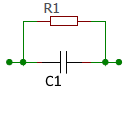# RC parallel circuit

Calculator and formulas for calculation of current and power of an RC parallel circuit

## Calculate RC parallel circuit

The calculator calculates current, power, impedance and reactance in the parallel circuit of a resistor and a capacitor.

RC parallel calculator

 Input Capacitor F mF µF nF pF Frequency Hz kHz MHz GHz Resistor mΩ Ω kΩ MΩ Voltage mV V kV Decimal places 0 1 2 3 4 6 8 10 Result Reactance XC Impedance Z Resistor current IR Capacitor current IC Total current I Real power P Reactive power Q Apparent power S Phase angle φ## Formulas for parallel parallel circuit

The total resistance of the RC series circuit in the AC circuit is called Impedance Z. Ohm's law applies to the entire circuit.

Current and voltage are in phase at the ohmic resistance. At the capacitive reactance of the capacitor, the voltage lags the current by −90 °.

The total current I is the sum of the geometrically added partial currents. For this purpose, both partial flows form the legs of a right triangle. Its hypotenuse corresponds to the total current I. The resulting triangle is called the current triangle or vector diagram of the currents.

### Current triangle

 $$\displaystyle I=\sqrt{{ I_R }^2+{I_C}^2}$$

 $$\displaystyle I$$ Total current $$\displaystyle I_R$$ Current through the resistor $$\displaystyle I_C$$ Current through the capacitor

### Conductance triangle

In parallel circuit, the partial currents behave like the conductance values of resistances.

 $$\displaystyle y=\sqrt{G^2+{B_C}^2}$$

 $$\displaystyle G$$ Conductance [1/R] $$\displaystyle B_C$$ Susceptance [1/XC] $$\displaystyle Y$$ Admittance [1/Z]

### Resistance triangle

 $$\displaystyle Z=\frac{X_C · R}{\sqrt{R^2+{X_C}^2}}$$

 $$\displaystyle X_C$$ Capacitive reactance $$\displaystyle R$$ Effective resistance $$\displaystyle Z$$ Impedance

### Power triangle

 $$\displaystyle S=\sqrt{P^2+Q^2}$$

 $$\displaystyle S$$ Apparent power $$\displaystyle P$$ Real power $$\displaystyle Q$$ Reactive power

### Real power

 $$\displaystyle P=U · I_R$$

 $$\displaystyle P$$ Real power $$\displaystyle U$$ Voltage $$\displaystyle I_R$$ Current through the resistor

### Reactive power

 $$\displaystyle Q=U · I_C$$

 $$\displaystyle Q$$ Reactive power $$\displaystyle U$$ Voltage $$\displaystyle I_C$$ Current through the capacitor